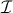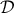Courbes multiples primitives et déformations de courbes lisses .  preprint (2011), à paraître dans les Annales de la Faculté des Sciences de Toulouse.

A primitive multiple curve is a Cohen-Macaulay scheme Y over such that the reduced scheme C = Y red is a smooth curve, and that Y can be locally embedded in a smooth surface. In general such a curve Y cannot be embedded in a smooth surface. If Y is a primitive multiple curve of multiplicity n, then there is a canonical filtration  C = C1Cn = Y  such that Ci is a primitive multiple curve of multiplicity i. The ideal sheafC of C in Y is a line bundle on Cn-1.

Let T be a smooth curve and t0 T a closed point. LetT be a flat family of projective smooth irreducible curves, and C =t0. Then the n-th infinitesimal neighbourhood of C inis a primitive multiple curve Cn of multiplicity n, embedded in the smooth surface, and in this caseC is the trivial line bundle on Cn-1. Conversely, we prove that every projective primitive multiple curve Y = Cn such thatC is the trivial line bundle on Cn-1 can be obtained in this way.